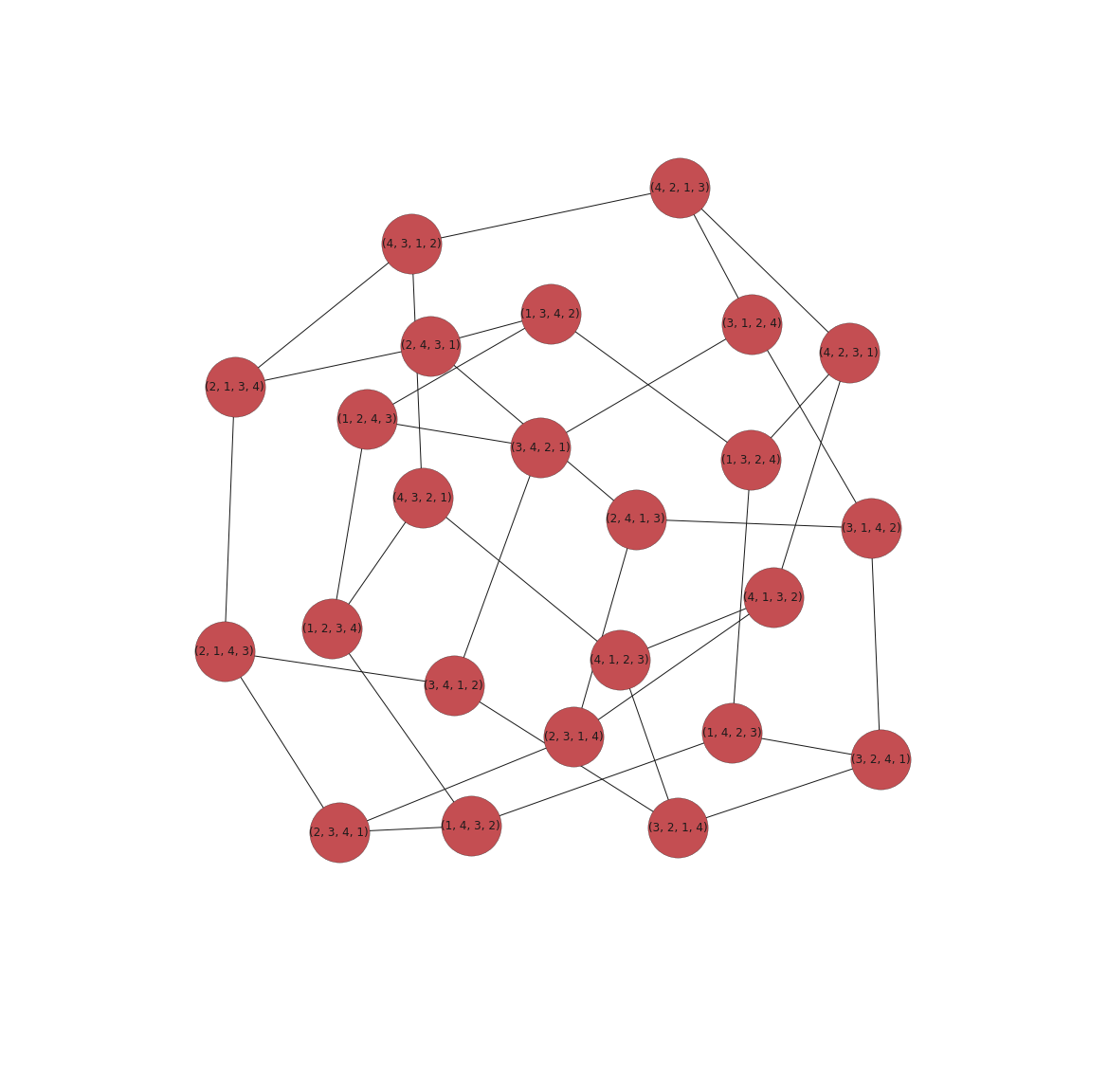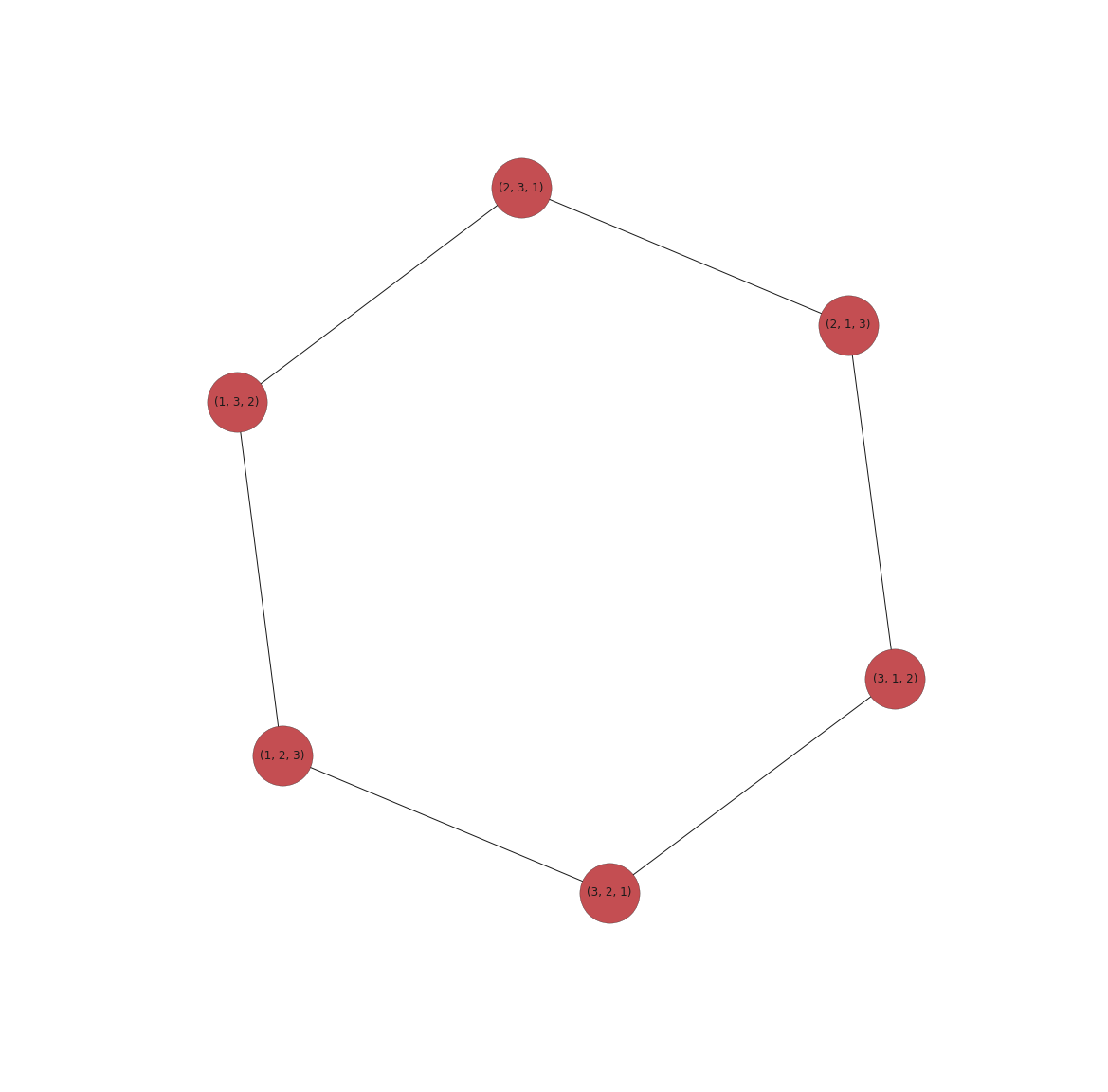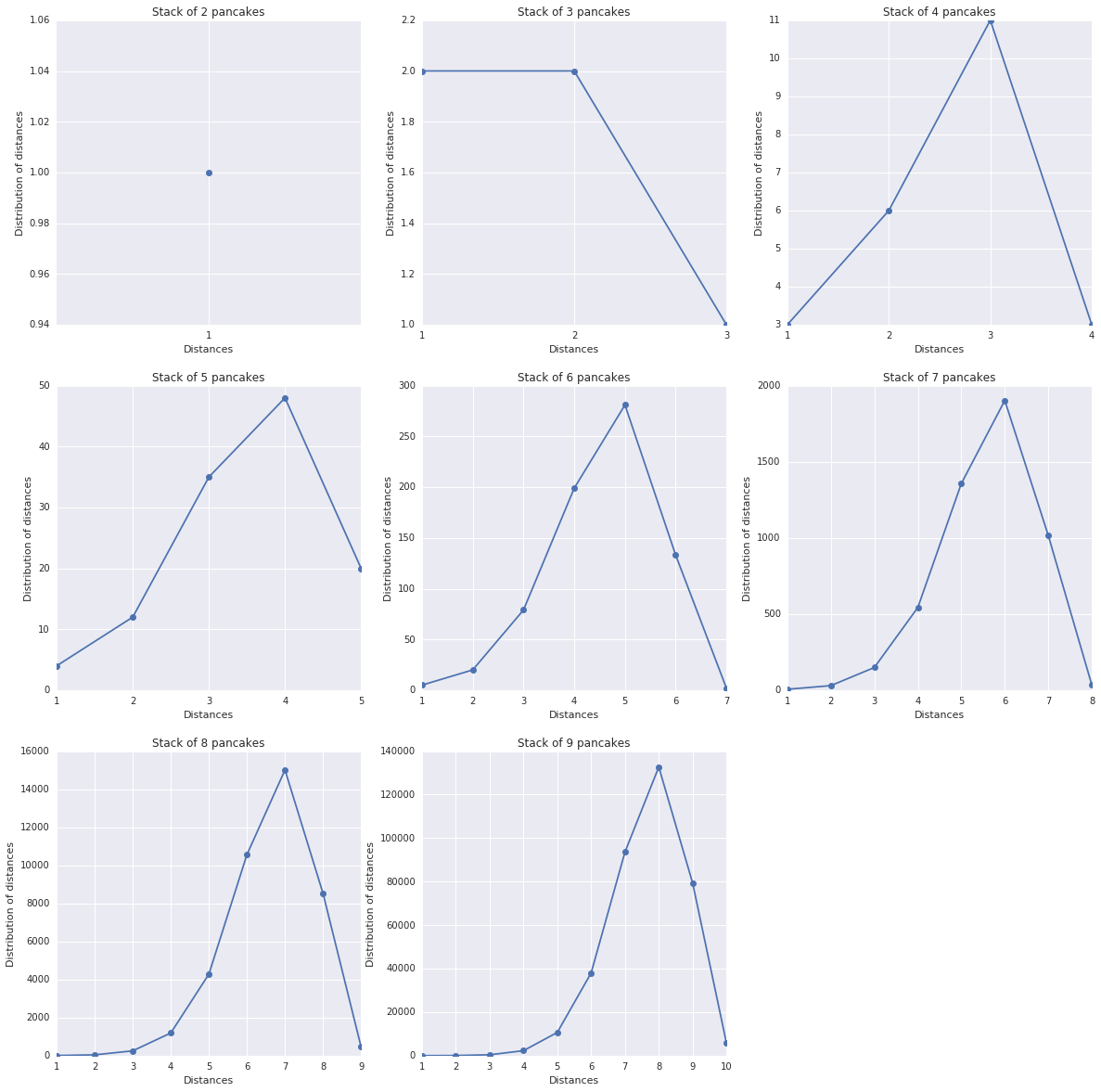# Investigating pancake stacks¶

At the last MathsJam event in Lund, we decided to work on the pancake problem. Statement:

A stack of different sized pancakes can be sorted into size order by repeatedly inserting a spatula underneath one of the pancakes, and flipping the top section over.

1. What's the most flips you might need to sort a stack of four different sized pancakes?
2. With 6 pancakes, exactly two stacks exist that need 7 flips. Can you find one?

## First thoughts¶

We first interpreted most flips by worst case scenario. Then an algorithm that works to re-order the stack by pancake size is to:

1. Put the spatula under the biggest pancake
2. Flip. The big pancake is at the top
3. Flip everything from the bottom. The big pancake is at the correct position (bottom)
4. Repeat by ignoring the large pancake just sorted

Wikipedia says that this approach is like a selection sort. And it is: find the largest pancake and put it at the top and then at the bottom.

It looks optimal in the most number of flips, meaning that we will always be able to sort the stack by proceeding like this. But it is not necessary the optimal way of flipping (finding the most flips).

So we decided to investigate what happens with different stack sizes and some programming.

## With a graph¶

The stack of pancakes can be represented as a list of integers, all different. A stack of 4 pancakes will be [3, 2, 4, 1]. Its corresponding sorted stack is [1, 2, 3, 4].

The number of permutations of 4 numbers is $4! = 24$. More generally the number of permutations for a stack of size $n$ is $n!$.

To model the graph:

• the nodes are the permutations. There will be 24 nodes for the stack of size 4.
• the edges represent the transitions between the permutations.

To construct the graph:

• list all the permutations.
• for each permutations, flip from every positions in the stack and add the new permutation as a neighbor of the current one.

Once the graph is constructed, we can plot it (if the stack size isn't too big). Then, traverse the graph (BFS) from the node [1, 2, 3, 4], to produce the list of distances between this node (sorted) and all the others. Then the most flips is the biggest of all the distances.

In :
# Imports
import networkx as nx

import matplotlib.pyplot as plt
%matplotlib inline
import seaborn as sns
sns.set(color_codes=True)

from itertools import permutations
from collections import Counter

In :
def plot_graph(g):
'''
Plot the networkx graph with custom parameters so it is not
too small. To be used for small values of n
'''
plt.figure(figsize=(20,20), dpi=80)
nx.draw_networkx(g, node_shape='o', node_size=4000)
plt.axis('off')
plt.show()

def gen_graph(n):
'''
Generate the graph of permutations with edges connecting
two permutations linked by a flip
'''
A = list(range(1, n + 1))
ps = list(permutations(A))
source = tuple(sorted(A))
g = nx.Graph()

for p in ps:
for i in range(n-1):
p2 = p[0:i] + p[i:][::-1]

return g, source


## Question 1¶

What's the most flips you might need to sort a stack of four different sized pancakes?

To answer the first question, we calculate the distances between the sorted permutation (final state) and all the others, for a stack of 4 pancakes.

In :
def print_max_dist(dists, n):
max_dist = max(dists, key=lambda x: dists[x])
print('Max number of flips for', n, 'pancakes is for the stack', max_dist, 'with', dists[max_dist])

def calc_distances(n, plot=True):
g, source = gen_graph(n)
dists = nx.shortest_path_length(g, source=source)

if plot:
# Plot the graph to visually check the connections
plot_graph(g)

return g, source, dists

In :
# Study the case for a stack of 4 pancakes
g, source, dists = calc_distances(4)
print_max_dist(dists, 4)Max number of flips for 4 pancakes is for the stack (3, 1, 4, 2) with 4


Event for a small number of stacks, the graph is already difficult to read.

Let's look at the case for 3 pancakes before moving on to the $2^{nd}$ question.

In :
# Study the case for a stack of 3 pancakes this time
g, source, dists = calc_distances(3)
print_max_dist(dists, 3)Max number of flips for 3 pancakes is for the stack (2, 1, 3) with 3


For a stack of 3 pancakes, [2, 1, 3] is the permutation that is the furthest from [1, 2, 3], as show on the graph.

We can now answer the second question by just changing the stack size.

## Question 2¶

With 6 pancakes, exactly two stacks exist that need 7 flips. Can you find one?

In :
# Study the case for a stack of 6 pancakes this time
# Do not plot the graph (too big)
g, source, dists = calc_distances(6, False)
print_max_dist(dists, 6)

stacks = [k for k, v in dists.items() if v == 7]
print('The stacks with 7 flips with the flips operations are:')
print()
for s in stacks:
print('-', s)
path = nx.shortest_path(g, source=s, target=source)
for i, node in enumerate(path):
print('  ', str(i) + '.', node)
print()

Max number of flips for 6 pancakes is for the stack (5, 3, 6, 1, 4, 2) with 7
The stacks with 7 flips with the flips operations are:

- (5, 3, 6, 1, 4, 2)
0. (5, 3, 6, 1, 4, 2)
1. (2, 4, 1, 6, 3, 5)
2. (2, 4, 5, 3, 6, 1)
3. (1, 6, 3, 5, 4, 2)
4. (1, 6, 3, 2, 4, 5)
5. (1, 6, 5, 4, 2, 3)
6. (1, 6, 5, 4, 3, 2)
7. (1, 2, 3, 4, 5, 6)

- (4, 6, 2, 5, 1, 3)
0. (4, 6, 2, 5, 1, 3)
1. (3, 1, 5, 2, 6, 4)
2. (3, 4, 6, 2, 5, 1)
3. (1, 5, 2, 6, 4, 3)
4. (1, 5, 2, 3, 4, 6)
5. (1, 5, 6, 4, 3, 2)
6. (1, 2, 3, 4, 6, 5)
7. (1, 2, 3, 4, 5, 6)



## Investigating more¶

It would be interesting to know how the number of flips (and its distribution) evolves with the number of pancakes. Unfortunately, the number of permutations starts to be pretty big for stacks with more than 10 pancakes, as $10! = 3628800$.

In :
LIMIT = 10
cnts = []
for n in range(2, LIMIT):
g, source = gen_graph(n)
dists = nx.shortest_path_length(g, source=source)
values = [d for d in dists.values() if d != 0]
cnt = Counter(values).most_common()
cnts.append(cnt)
print(n, ':', cnt)

2 : [(1, 1)]
3 : [(1, 2), (2, 2), (3, 1)]
4 : [(3, 11), (2, 6), (1, 3), (4, 3)]
5 : [(4, 48), (3, 35), (5, 20), (2, 12), (1, 4)]
6 : [(5, 281), (4, 199), (6, 133), (3, 79), (2, 20), (1, 5), (7, 2)]
7 : [(6, 1903), (5, 1357), (7, 1016), (4, 543), (3, 149), (8, 35), (2, 30), (1, 6)]
8 : [(7, 15011), (6, 10561), (8, 8520), (5, 4281), (4, 1191), (9, 455), (3, 251), (2, 42), (1, 7)]
9 : [(8, 132697), (7, 93585), (9, 79379), (6, 38015), (5, 10666), (10, 5804), (4, 2278), (3, 391), (2, 56), (1, 8)]

In :
plt.figure(figsize=(20,20), dpi=80)
fig_dims = (3, 3)

for i, cnt in enumerate(cnts):
cnt = sorted(cnt, key=lambda x: x)
x, y = [v for v in cnt], [v for v in cnt]
plt.subplot2grid(fig_dims, (i // 3, i % 3))
plt.title('Stack of ' + str(i + 2) + ' pancakes')
plt.xticks(x)
plt.xlabel('Distances')
plt.ylabel('Distribution of distances')
plt.plot(x, y, 'o-')Not bad, but it would be nice to know what happens for even bigger stacks of pancakes!